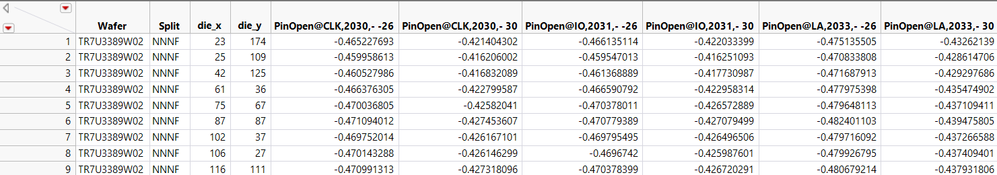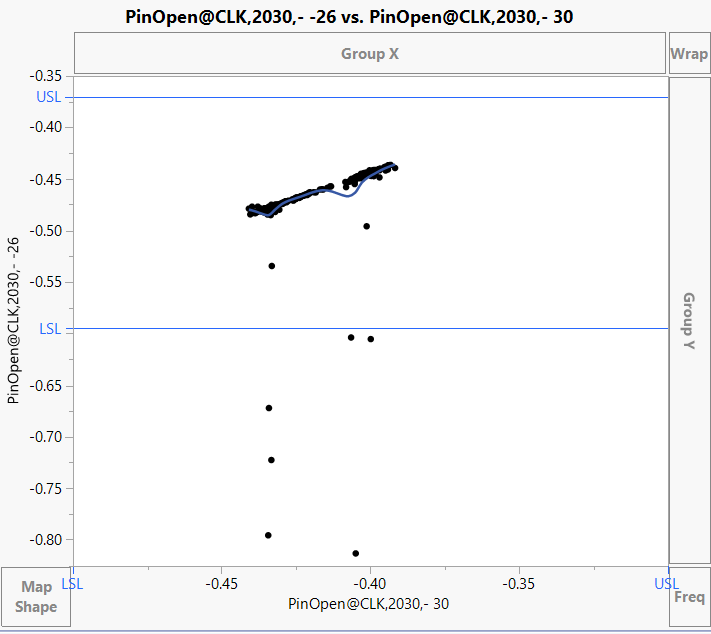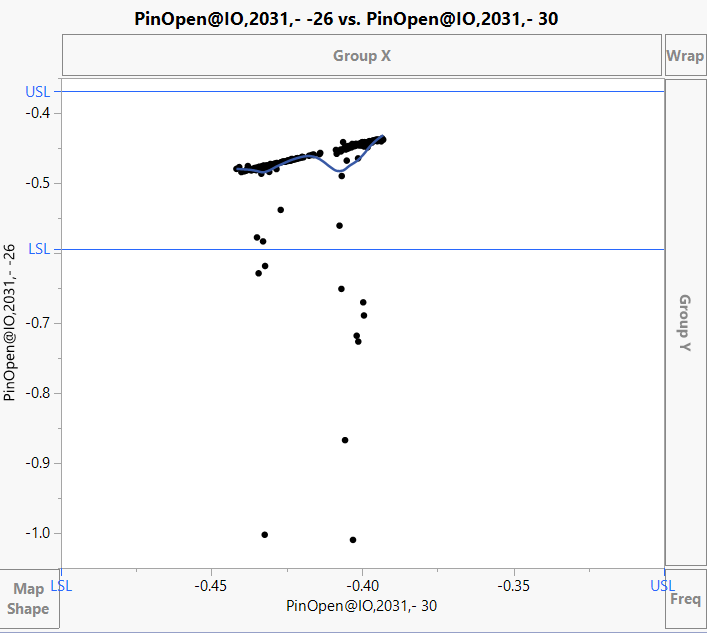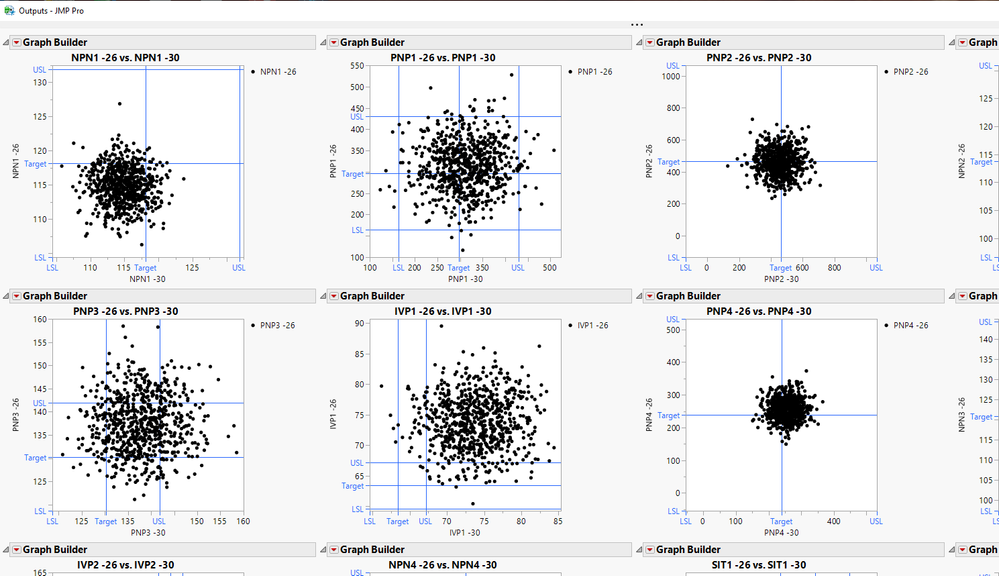Choose Language Hide Translation Bar
Highlighted

## Fit Y by X plot or correlation plot for multiple params

I want to do Fit Y by X plot for multiple parameters for 2 groups of data like below. If i only want to perform 1 params, it is pretty straight forward. I will just need to split the temp from row to column, and manually choose param1 temp1 vs param1 temp2. But if i have 100 parameters, it seem not so practically. Is there any other systematic or easy way to achieve this?

 Temp param1 param2 param3 paramx temp1 xx xx xx xx temp1 xx xx xx xx temp1 xx xx xx xx temp1 xx xx xx xx temp2 xx xx xx xx temp2 xx xx xx xx temp2 xx xx xx xx temp2 xx xx xx xx

After split the table something like this.1 ACCEPTED SOLUTION

Accepted Solutions
Highlighted

## Re: Fit Y by X plot or correlation plot for multiple params

I am not aware of a direct way to do what you want, however the script below will do it.  It is using the Semiconductor Capability data table from the JMP Sample Data Tables.  I think the code is simple enough to work through your use of it.

``````Names Default To Here( 1 );
// open the semiconductor data table and add a Temp column to it
// to mimic the data table you are using
dt = Open( "\$SAMPLE_DATA\semiconductor capability.jmp" );
dt << New Column( "Temp", nominal, formula( If( Row() < N Rows( dt ) / 2, -26, -30 ) ) );

// Create a list of all of the parameters
colList = dt << get column names( continuous, string );

// Turn on the Show Limits for all of the columns
For( i = 1, i <= N Items( colList ), i++,
spec = Column( dt, colList[i] ) << get property( "spec limits" );
insert into(spec,expr(show limits(1)));
column( dt, colList[i] ) << set property("spec limits", eval(spec));
);

// Create the split data table
dtSplit = dt << Split(
Split By( :Temp ),
Split( Eval( colList ) ),
Remaining Columns( Drop All ),
Sort by Column Property
);

// Create an output window to place the graphs into
nw = New Window( "Outputs", lub = Lineup Box( N Col( 4 ) ) );

// Since the columns desired are side by side, loop across
// the columns and create the graphs and add them to the
// output window
For( i = 1, i <= N Cols( dtSplit ), i = i + 2,
lub << append(
Graph Builder(
Size( 350, 350 ),
Show Control Panel( 0 ),
Variables( X( As Column( dtSplit, i ) ), Y( As Column( dtSplit, i + 1 ) ) ),
Elements( Points( X, Y, Legend( 5 ) ) )
)
)
);``````Jim
5 REPLIES 5
Highlighted

## Re: Fit Y by X plot or correlation plot for multiple params

I interpret your request to mean that you have many data columns to be used in both the X and Y role when making a scatter plot. The Multivariate platform automatically produces a matrix of such scatter plots. Enter all the variables in the Y role and you will get all possible scatter plots, two variables at a time.

Another interpretation, though, is that you want the same scatter plot but split the data by "Temp." If so, then put Temp in the By role when you launch the Bivariate platform through the Fit Y by X dialog box.

Finally, if the need is to interactively explore the scatter plot with different data columns in the X or Y role, then use the Column Switcher feature.

Hopefully one of these suggestions will be helpful or prompt clarification about what you want to do.

Learn it once, use it forever!
Highlighted

## Re: Fit Y by X plot or correlation plot for multiple params

Thanks for reply. Either of your option fit to my requirement. Maybe my explanation is not clear enough in the first place.

Multivariate indeed can do 1 vs N parameter in the same time. But it is not practically if you want to do hundreds of params. And i also don't need 1 vs N parameter correlation. I only need 1 to 1 parameter correlation. Your second option is actually suggest doing Param1 vs Param2 group by Temp, which is also not my idea.

What i really want is ..

Chart1 : Y-axis will be temp1 param1 & X axis will be temp2 param1.

Chart2: Y-axis will be temp1 param2 & X axis will be temp2 param2.

and so on.... for 100 hundreds params

Highlighted

## Re: Fit Y by X plot or correlation plot for multiple params

I am not aware of a direct way to do what you want, however the script below will do it.  It is using the Semiconductor Capability data table from the JMP Sample Data Tables.  I think the code is simple enough to work through your use of it.

``````Names Default To Here( 1 );
// open the semiconductor data table and add a Temp column to it
// to mimic the data table you are using
dt = Open( "\$SAMPLE_DATA\semiconductor capability.jmp" );
dt << New Column( "Temp", nominal, formula( If( Row() < N Rows( dt ) / 2, -26, -30 ) ) );

// Create a list of all of the parameters
colList = dt << get column names( continuous, string );

// Turn on the Show Limits for all of the columns
For( i = 1, i <= N Items( colList ), i++,
spec = Column( dt, colList[i] ) << get property( "spec limits" );
insert into(spec,expr(show limits(1)));
column( dt, colList[i] ) << set property("spec limits", eval(spec));
);

// Create the split data table
dtSplit = dt << Split(
Split By( :Temp ),
Split( Eval( colList ) ),
Remaining Columns( Drop All ),
Sort by Column Property
);

// Create an output window to place the graphs into
nw = New Window( "Outputs", lub = Lineup Box( N Col( 4 ) ) );

// Since the columns desired are side by side, loop across
// the columns and create the graphs and add them to the
// output window
For( i = 1, i <= N Cols( dtSplit ), i = i + 2,
lub << append(
Graph Builder(
Size( 350, 350 ),
Show Control Panel( 0 ),
Variables( X( As Column( dtSplit, i ) ), Y( As Column( dtSplit, i + 1 ) ) ),
Elements( Points( X, Y, Legend( 5 ) ) )
)
)
);``````Jim
Highlighted

## Re: Fit Y by X plot or correlation plot for multiple params

Thanks Nelson. I think it could be the closed solution that i can get.
Highlighted